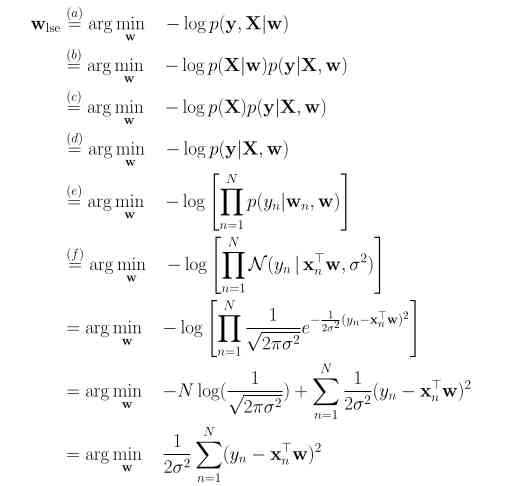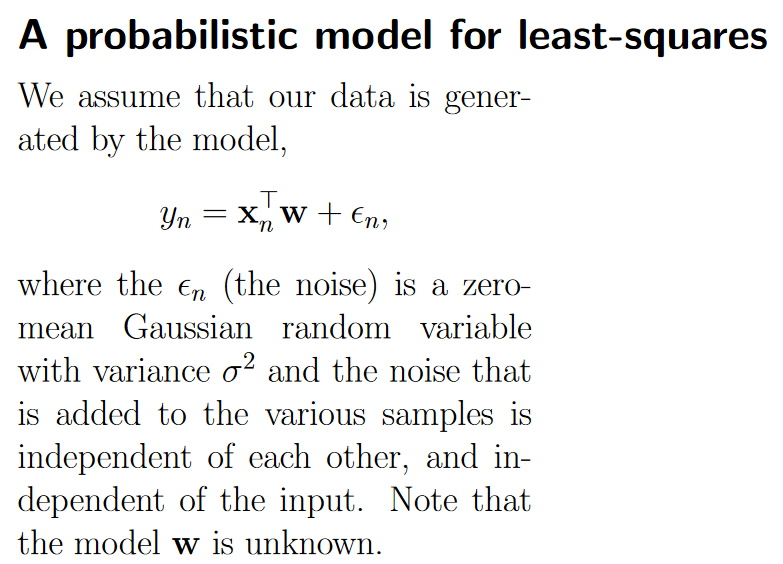### Linear Regression Assumption

Hi,
I don't understand why, in a linear regression, the gaussian assumption on the target is not equal to the gaussian assumption on the error. See question 1 of the exam.

If we don't assume that y follows a normal distribution, for example, let's assume that y follows a poison distribution, how could we derive MSE from the maximum log-likelihood?

From the lecture notes, the assumption from step e to step f is that y is normally distributed.

Could we really interpret least-squares linear regression as MLE assuming ANY distribution??The assumption was not that y is normally distributed but the following:Thank you Karel. I completely agree that the noise should have zero mean. However, I also think that in order to derive MSE from MLE, we need to assume that y also follows a normal distribution.

Do you know if the error is gaussian, does that make y also gaussian?

I would like to understand this more. I'm guessing there might be some steps missing in the derivation.

Is step e, the sum of two gaussians?

Yes I also was a bit confused by this question. By assuming the above model, we are still assuming y is a normal distribution with mean $$x_{n}^{T}w$$ and variance $$\sigma^{2}$$ due to the noise. Would like to understand what the distinction there is :)

Hi,

I think the confusion comes from the fact that $$y$$ given $$x$$ has a normal distribution $$\mathcal{N}( x^T w, \sigma^2)$$. However $$y$$ can be very different from a Gaussian (the total distribution can be very different from the conditional distributions).

For instance assume $$d = 1$$ and $$x = +1$$ w.p $$1/ 2$$ and $$- 1$$ otherwise, then if the noise is $$\mathcal{N}( 0, \sigma^2)$$ and independent of $$x$$ we get that : $$p( y | x = \pm 1) = \mathcal{N}( \pm w, \sigma^2 )$$ and
$$p(y) = 0.5 \ \mathcal{N}( w, \sigma^2 ) + 0.5 \ \mathcal{N}( - w, \sigma^2)$$, which is not Gaussian.

Let me know if this is clear. Best.

Scott

Page 1 of 1